# How To Add Text Box To a Chart In Excel?

Sometime we may want to place a textbox above each bar of a bar graph to provide additional information. Such textboxes help the users understand the graph quickly and analyse the data in a better way. Most of the time, we use such textboxes to highlight the highest and lowest points in a graph, for example, highest and lowest sales numbers. Read through this tutorial to find out how you can follow a simple process to add a textbox above each bar of a bar graph.

## Adding Text Box To a Chart In Excel

Here we will first create a bar graph from a given set of data and thereafter, we will insert a textbox in the chart to highlight the highest sales figure.

### Step 1

To create a bar graph, select the data and select "2d bar graph" from the Graph section under the "Insert" menu, as shown in the following image -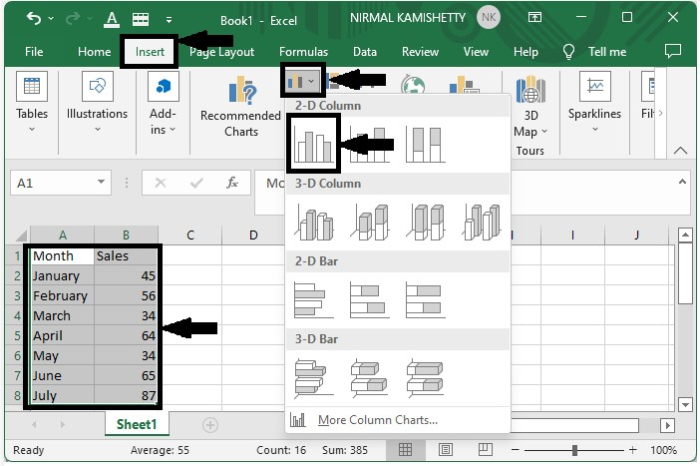It will create a bar graph, as shown below -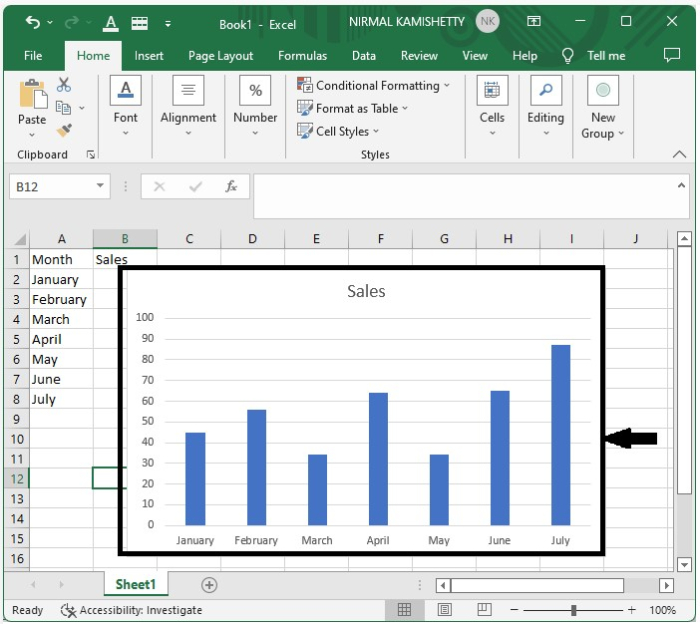### Step 2

To create a textbox above the bar, click the Add Text button present under the Insert menu of the toolbar.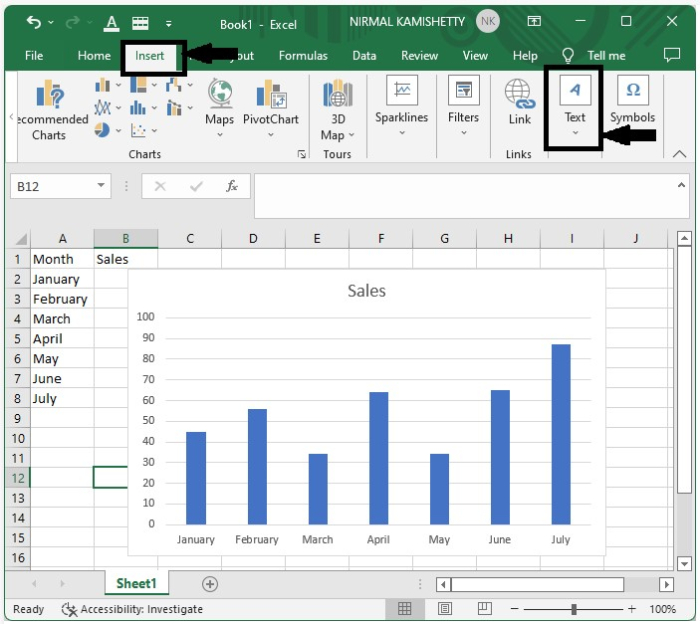This will create a textbox which we can place above the bar of the graph, just by moving the box exactly above the graph.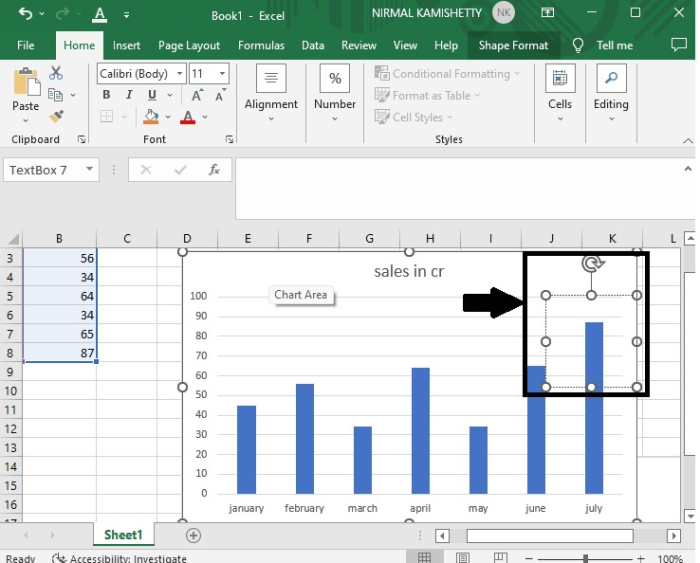### Step 3

After creating the textbox, add some text to it (in our case, we have used the label "highest" inside the textbox), and then you can move the textbox around and place it exactly over the tallest bar to highlight the highest sales figure.

The final result will appear like the image shown below -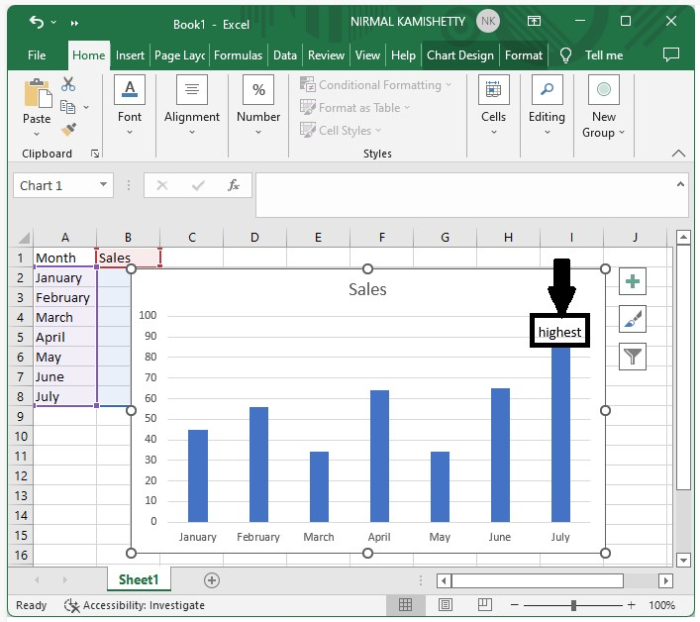You can format the textbox, for example, change the font typeface, font size, colour and also the border of the text, by right-clicking on the text and selecting the "Format" option. You can use this option to customize the field colour, field outline, text colour, text font and many more.

## Conclusion

In this tutorial, we used a simple example to demonstrate how you can add a textbox in a bar graph in Excel to highlight a particular set of data. You can follow the same process to add multiple textboxes over different bars in a bar chart.